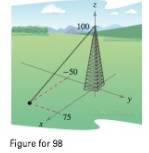Chapter 11.2, Problem 100E

Chapter
Section
Textbook Problem

# Tower Guy Wire The guy wire supporting a 100-foot lower has a tension of 550 pounds. Using the distance shown in the figure, write the component form of the vector F representing the tension in the wire.To determine
The component form of the vector F that represents the tension in the wire.

Explanation

Given: The height of the tower is 100-foot and the tension 550 pounds as shown in figure below

Explanation: Consider the point P on the groundas seen on the figure, the co ordinates of P are (75, -50, 0) and let the top of the tower be Q and coordinates of it be (0, 0, 100).

PQ=075,0+50,1000=75,50,100

Since F is parallel to  PQ . So,

F=c.PQ, where  c be a scalar

Therefore,

PQ=075,0+50,1000=75,50,100

And

F</

### Still sussing out bartleby?

Check out a sample textbook solution.

See a sample solution

#### The Solution to Your Study Problems

Bartleby provides explanations to thousands of textbook problems written by our experts, many with advanced degrees!

Get Started

#### Solve the equations in Exercises 126. 1x211+1=0

Finite Mathematics and Applied Calculus (MindTap Course List)

#### In problems 45-48, determine a value for that makes each statement true. 48.

Mathematical Applications for the Management, Life, and Social Sciences

#### Multiply. (34x)(25x)

Trigonometry (MindTap Course List)

Study Guide for Stewart's Multivariable Calculus, 8th